## Periodic Classification of Elements Class 10 MCQs Questions with Answers

Periodic Classification Of Elements Class 10 MCQ Question 1.
Which of the following statements) about the modern periodic table are incorrect?
(I) The elements in the modern periodic table are arranged on the basis of their decreasing atomic numbers.
(II) The elements in the modern periodic table are arranged on the basis of their increasing atomic masses.
(III) Isotopes are placed in adjoining group(s) in the periodic table.
(IV) The elements in the modern periodic table are arranged on the basis of their increasing atomic number.
(a) Only (I)
(b) (I), (II), and (III)
(c) (I), (II), and (IV)
(d) Only (IV)
(b) (I), (II), and (III)

Explanation: The modern periodic table is a tabular arrangement of elements in increasing order of their atomic number, which is why isotopes are placed at the same position. Hence, only (III) is correct.

Periodic Table Class 10 Icse MCQ Question 2.
Which of the following statements about the modern periodic table is correct?
(a) It has 18 horizontal rows known as periods.
(b) It has 7 vertical columns rows known as periods.
(c) It has 18 vertical columns known as groups.
(d) It has 7 horizontal rows known as groups.

MCQ On Periodic Classification Of Elements Question 3.
An element X is forming the acidic oxide. Its most probable position in the modern periodic table is:
(a) Group 1 and Period 3
(b) Group 16 and Period 3
(c) Group 17 and Period 3
(d) Group 2 and Period 3Class 10 Science Chapter 5 MCQ Question 4.
Which of the given elements A, B, C, D and E with atomic numbers 2, 3, 7,10 and 30 respectively belong to the same period?
(a) A, B, C
(b) B, C, D
(c) A, D, E
(d) B, D, E

MCQ On Periodic Classification Of Elements Class 10 Pdf With Answers Question 5.
On the basis of the electronic configuration of x|, the group number and period of the element ‘X is:
(a) Group 15 period 2
(b) Group 13 period 2
(c) Group 9 period 5
(d) Group 13 period 5
(b) Group 13 period 2

Explanation: From X95, we can have the information as:
X — element
5 — Atomic number
9 — Mass number
The electronic configuration of X is 2, 3.

Group number is found out on the basis of valance electrons. Valence electrons are 3 so group number is 10 + 3 = 13.

The period can be found out by knowing the number of shells. Since the number of shells is 2 so it belongs to period 2.

Valence Electron Calculator – This free calculator provides you with free information about Valence Electron.

Periodic Classification Of Elements MCQ Question 6.
The elements A, B, C, D and E have atomic number 9, 11, 17, 12 and 13 respectively.
Which pair of elements belongs to the same group?
(a) A and B
(b) B and D
(c) A and C
(d) D and EMCQ Of Periodic Classification Of Elements Question 7.
An element ‘X‘ with atomic number 11 forms a compound with element ‘V with atomic number 8. The formula of the compound formed is:
(a) XY
(b) X2Y
(c) XY2
(d) X2Y3
(b) X2Y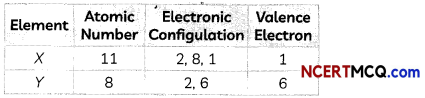Element X can lose one electron and has a configuration like that of Neon.
X → X+ + e
Element Y can gain two electrons to acquire noble gas configuration like that of Neon:
Y + 2e → Y2
Or we can write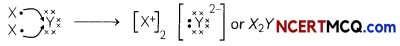Periodic Table MCQ Questions And Answers Question 8.
The positions of four elements A, B, C, and D in the modern periodic table are shown below.Which element is most likely to form an acidic oxide?
(a) A
(b) B
(c) C
(d) D

Chapter 5 Science Class 10 MCQ Question 9.
In Mendeleev’s periodic table, gaps were left for the elements to be discovered later. Which of the following element found a place in the periodic table later?
(a) Germanium
(b) Chlorine
(c) Oxygen
(d) Silicon

Class 10 Science Ch 5 MCQ Question 10.
Elements P, Q, R, and S have atomic numbers 11,15,17 and 18 respectively. Which of them are reactive non-metals?
(a) P and Q
(b) P and R
(c) Q and R
(d) R and S
(c) Q and RChapter 5 Class 10 Science MCQ Question 11.
An element which is an essential constituent of all organic compounds belongs to:
(a) Group 1
(b) Group 14
(c) Group 15
(d) Group 16

MCQs Of Periodic Classification Of Elements Question 12.
According to Mendeleev’s periodic law, the elements were arranged in the periodic table in the order of:
(a) Increasing atomic number
(b) Decreasing atomic number
(c) Increasing atomic masses
(d) Decreasing atomic masses
(c) Increasing atomic masses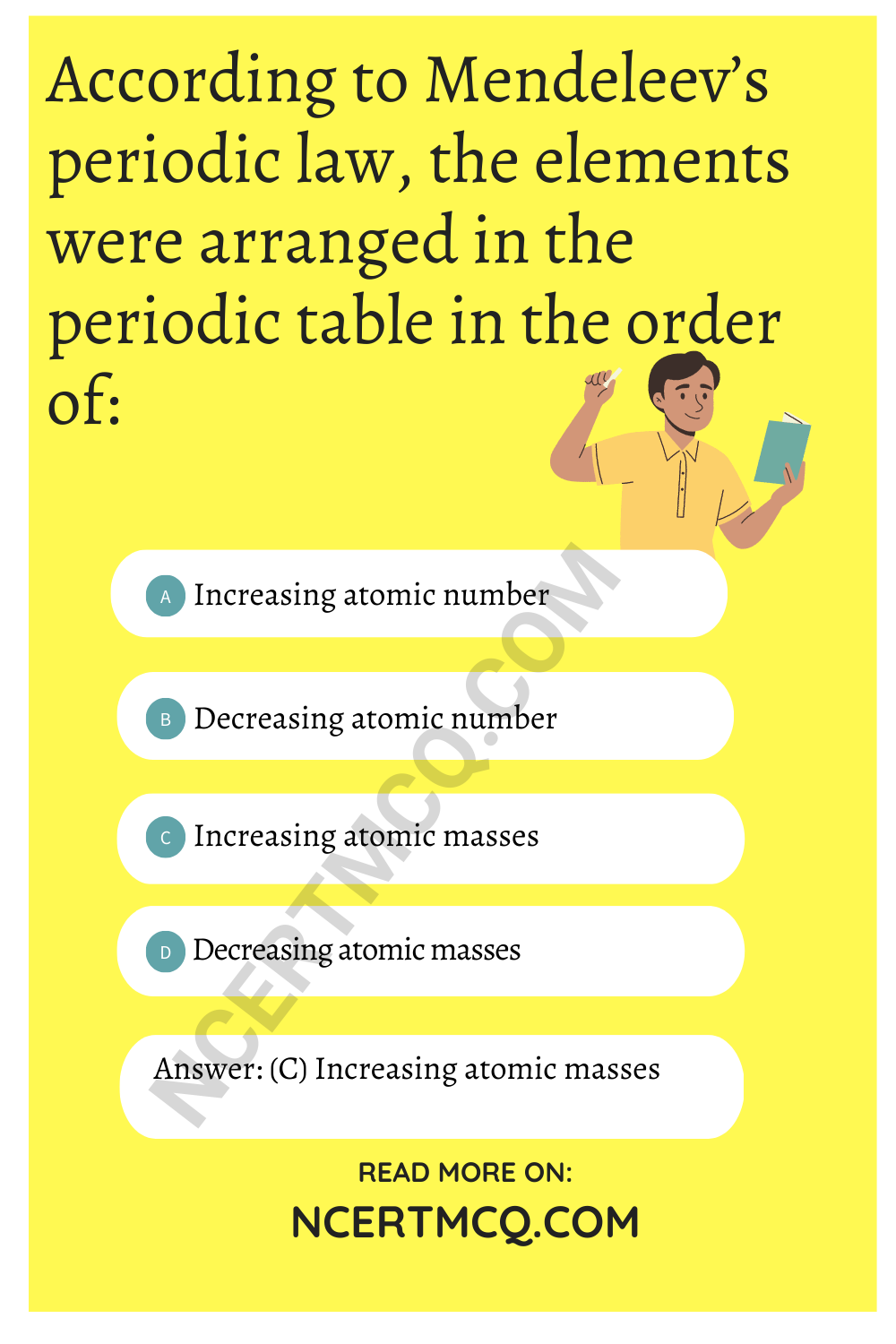Explanation: Mendeleev’s periodic law is an arrangement of elements in increasing order of their atomic masses as Mendeleev’s periodic law states that, the physical and chemical properties of elements are a periodic function of their atomic masses.

Class 10 Chapter 5 Science MCQ Question 13.
Which of the following is the outermost shell for elements of period 2?
(a) K shell
(b) L shell
(c) M shell
(d) N shell

MCQ Periodic Classification Of Elements Question 14.
Which of the following gives the correct increasing order of the atomic radii of O, F, and N?
(a) O, F, N
(b) N, F, O
(c) O, N, F
(d) F, O, N

Periodic Table Class 10 MCQ Question 15.
Arrange the following elements in the order of their decreasing metallic character Na, Si, Cl, Mg, Al:
(a) Cl > Si > Al > Mg > Na
(b) Na > Mg > Al > Si > Cl
(c) Na > Al > Mg > Cl > Si
(d) Al > Na > Si > Ca > Mg

Question 16.
Which among the following elements has the largest atomic radii?
(a) Na
(b) Mg
(c) K
(d) Ca
(c) K

Explanation: Na and Mg belong to the same period, whereas Na and K are in the same group. In a group from top to bottom, the atomic radius increases, whereas it decreases in a period from left to right. Hence, the order would be K > Na > Mg and K > Ca > Mg.

Related Theory:
Atomic radius represents the distance from the nucleus to the outer shell of an element.
The trend of the atomic radius in the periodic table:

1. decreases going from left to right of a period
2. increases going down a group.

Question 17.
The electronic configurations of three elements X, Y and Z are:
X : 2 Y: 2, 8, 7 Z : 2, 8, 2
Which of the following is correct regarding these elements?
(a) X is a metal
(b) Y is a metal
(c) Z is a non-metal
(d) Y is a non-metal and Z is a metal
(d) Y is a non-metal and Z is a metal.

Explanation: Element Y has 1 less electron to complete its octet which is a property of non-metals. On the other hand, element Z has 2 extra electrons to become stable which is a property of metals..

Question 18.
Which of the following elements would lose an electron easily?
(a) Mg
(b) Na
(c) K
(d) CaQuestion 19.
Which of the following elements does not lose an electron easily?
(a) Na
(b) F
(c) Mg
(d) Al
(b) F

Explanation: Flourine has 7 electrons in the outermost orbit. Its atom size is the smallest too. Since it is the most electronegative element, it does not lose an electron easily. Sodium has one valence electron, magnesium has 2 valence electrons, and aluminium has 3 valence electrons. All these metals lose electrons easily.

Related Theory
Metals can easily lose electrons and exhibit electropositive nature, whereas non-metals have a tendency to accept electrons and exhibit electronegative nature. Na, Al, and Mg are metals, whereas F is non-metal.

Question 20.
What type of oxide would Eka-aluminium form?
(a) EO3
(b) E3O2
(c) E2O3
(d) EO

Question 21.
The element with atomic number 14 is hard and forms acidic oxide and a covalent halide. To which of the following categories does the element belong?
(a) Metal
(b) Metalloid
(c) Non-metal
(d) Left-hand side element
(b) Metalloid

Explanation: Atomic number 14 (electronic configuration of 2,8,4) is for silicon element, which is a metalloid and exhibits properties of both metal and non-metal. It has 4 electrons in its valence shell, so it tends to form covalent acidic oxide.

Question 22.
Which one of the following depicts the correct representation of atomic radius (r) of an atom?(a) (I) and (II)
(b) (II) and (III)
(c) (III) and (IV)
(d) (I) and (IV)
(b) (II) and (III)

Explanation: The distance between the center of the nucleus and the outermost shell is known as atomic radius.

Question 23.
Which one of the following does not increase while moving down the group of the periodic table?
(b) Metallic character
(c) Valence
(d) Number of shells in an element

Question 24.
On moving from left to right in a period in the periodic table, the size of the atom:
(a) increases
(b) decreases
(c) does not change appreciably
(d) first decreases and then increases
(b) decreases

Explanation: As we move from left to right in the period of periodic table, the number of shell remains same but atomic number increases. So, the increase in nuclear charge which tends to pull electrons closer to the nuclers and reduces the size of the atoms.

Related Theory
The size of atom of an inert gas is bigger than that of the preceding halogen atom. The greater size of the inert gas atom in a period is due to the structural stability of its outermost shell consisting of an octet of electrons.Question 25.
Which of the following set of elements is written in order of their increasing metallic character?
(a) Be, Mg, Ca
(b) Na, Li, K
(c) Mg, Al, Si
(d) C, O, N
(a) Be, Mg, Ca.

Explanation: As we move down in a group, the metallic nature of elements increases. Be, Mg, and Ca are present in the same group (group 2 of the modern periodic table). Thus, Ca has the highest metallic character.

Question 26.
An element X makes an oxide with the formula X2O3. This element will be in the same group as:
(a) Na
(b) Mg
(c) Al
(d) Cl [Diksha]
(c) Al

Explanation: The answer is Al (aluminium). The element Al is in group II! of Mendeleev’s periodic table, in which the general formula of the oxides of elements is X2O3.

Question 27.
The two pairs of elements placed by Newlands in one slot are:
(a) Co, Ni and Ce, La
(b) Be, Mg and Co, Ni
(c) F, Cl, and Ce, La
(d) Zn, Sr, and Be, Mg

Question 28.
The order of metallic character of some elements is: N < P < As < Sb < Bi. Identify the most electronegative among these elements,
(a) N
(b) P
(c) Sb
(d) Bi
(a) N

Explanation: The less metallic an element, the more electro-negative it will be. Since electronegativity is the ability of an atom to gain electrons, whereas metallic character is related to the ability of atoms to lose electrons. As N is the least metallic of the given elements, it will be the most electronegative.

Question 29.
Four elements P, Q, R and S have number of valence electrons 2,3,5 and 7 respectively. A student noted down the Group number in Modern Periodic Table and their valency as: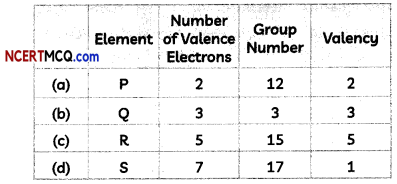Select the row containing the correct group number and valency.

Question 30.
Consider the elements Li, Be, B, C, N, O and F.
(I) The most electropositive element is F.
(II) The most electronegative element is F.
(III) The only metalloid is B.
(IV) The smallest atomic size is Li.
Read the statements given above and select the incorrect statements:
(a) Both (I) and (III)
(b) Both (I) and (IV)
(c) Both (II) and (III)
(d) Both (II) and (IV)
(b) Both (I) and (IV)

Explanation: Let us arrange the given elements according to their atomic number:
Li < Be < B < C < N < 0 < F.

All the above elements have two occupied shells and are therefore placed in the second group of the periodic table.

The most electropositive element is Li and the most electronegative element is F. This is because the tendency to lose electrons decreases as we go from left to right along a period. Also, as we go from left to right along a period, the atomic radius and hence the atomic size decreases because of the increase in effective nuclear charge experienced by the valence electrons. The only metalloid is B.Question 31.
Out of the elements F, Cl, Br and I, which is the most electronegative element?
(a) F
(b) Cl
(c) Br
(d) I
(a) F

Explanation: The electronegativity decreases as we move down a group because the ability to gain electrons decreases down a group. This is because of the decrease in the effective nuclear charge experienced by the valence electrons since a new shell is added in each group.

Since F, Cl, Br and I have 2, 3, 4 and 5 shells respectively, F is the most electronegative of these elements.

### Assertion Reasoning questions Class 10 Science Chapter 5

For the following questions, two statements are given-one labeled Assertion (A) and the other labeled Reason (R). Select the correct answer to these questions from the codes (a), (b), (c) and (d) as given below:
(a) Both (A) and (R) are true and (R) is the correct explanation of the assertion.
(b) Both (A) and (R) are true but (R) is not the correct explanation of the assertion.
(c) (A) is true but (R) is false.
(d) (A) is false but (R) is true.

Question 32.
Assertion (A): Potassium has a bigger atomic radius than lithium.
Reason (R): Atomic radius decreases along a period.

Question 33.
Assertion (A): Chemical properties of the elements belonging to the same group are the same.
Reason (R): Elements belonging to the same group possess the same number of valence electrons.
(a) Both (A) and (R) are true and (R) is the correct explanation of A.

Question 34.
Assertion (A): Nitrogen is a non-metal.
Reason (R): Nitrogen has 5 valence electrons.
(a) Both (A) and (R) are true and (R) is the correct explanation of (A)

Explanation: Non-metals are the elements that form negative ions by gaining electrons.

Electronic configuration of N = 2 5
k = 2
L = 5
It needs 3 electrons to complete its octet and achieve the same configuration as that of the inert gas neon.
N + 3e→ N3-

Question 35.
Assertion (A): Newland’s law of Octaves worked well with lighter elements only.
Reason (R): Newland assumed that only 56 elements existed in nature.Question 36.
Assertion (A): A correct position could not be assigned to hydrogen in Mendeleev’s periodic table.
Reason (R): Mendeleev Periodic Law states that ‘the properties of elements are the periodic function of their atomic numbers.
(c) (A) is true but (R) is false

Explanation: A correct position could not be assigned to hydrogen in the periodic table. The electronic configuration of hydrogen resembles that of alkali metals. Like alkali metals, hydrogen combines with halogens, oxygen and sulfur to form compounds having similar formulae.

On the other hand, just like halogens, hydrogen also exists as diatomic molecules and it combines with metals and non-metals to form covalent compounds.

Moreover, Mendeleev’s Periodic Law states that ‘the properties of elements are the periodic function of their atomic masses’.

Question 37.
Assertion (A): The elements Sodium (Na), Magnesium (Mg), Aluminium (Al) belong to the same group in Modern Periodic Table.
Reason (R): Atoms of different elements with the same number of occupied shells are placed in the same period.

Question 38.
Assertion (A): Calcium (Atomic No. 20) is more metallic than Potassium (Atomic No. 19).
Reason (R): The tendency to lose electrons decreases as we move from left to right in a period.
(c) (A) is false but (R) is true.

Explanation: Potassium (Atomic No. 19) is more metallic than Calcium (Atomic No. 20) as the tendency to lose electrons decreases as we move from left to right in a period.

The electronic configuration of Potassium is 2, 8, 8, 1 and that of Calcium is 2, 8, 8, 2. Therefore, the effective nuclear charge acting on the valence electrons of Calcium will be more than that of Potassium.

(Competency Based Questions (CBQs))

Question 1.
Sulphur is a multivalent non-metal, abundant, tasteless and and odorless. In its native form sulphur is a yellow crystalline solid. In nature it occurs as the pure element or as sulphide and sulphate minerals.Sulphur dioxide or sulphur dioxide is the chemical compound with theformula SO2. It is a toxic gas responsible for the smell of burnt matches. It is released naturally by volcanic activity and is produced as a by product of copper extraction and the burning of fossil fuels contaminated with sulphur compounds.
Identify the group and period of the Modern Periodic Table to which Sulphur belongs. Write any two properties exhibited by sulphur.
The atomic number of sulphur is 16. It’s electronic configuration is (2, 8, 6). It belongs to group 16 and period 3 of the Modern Periodic Table.

Two properties exhibited by sulphur are allotropy and catenation.Question 2.
Around the year 1800, only 30 elements were known. Dobereiner in 1817 and Newlands in 1866 tried to arrange the then known elements and framed laws which were rejected by the scientists. Even after the rejection of the proposed laws, many scientists continued to search for a pattern that correlated the properties of elements with their atomic masses.

The main credit for classifying elements goes to Mendeleev for his most important contribution to the early development of a Periodic table of elements wherein he arranged the elements on the basis of their fundamental property, the atomic mass and also on the similarity of chemical properties. The formulae of their hydrides and oxides were treated as basic criteria for the classification of the elements. However, Mendeleev’s classification also had some limitations as it could not assign the position to isotopes. He also left some gaps in the periodic table.
(A) State Mendeleev’s Periodic Law.
Mendeleev’s Periodic Law: It states that the properties of elements are the periodic function of their atomic masses.

Related Theory
Mendeleev concluded that when elements are arranged in order of increasing atomic masses, similar properties of element repented after a definite gap of atomic masses. This repetition of similar properties after a definite gap of atomic masses is also called periodicity in properties.

(B) Why did Mendeleev leave some gaps in the Periodic table?

(C) If the letter ‘R‘ was used to represent any of the elements in the group, then the hydride and oxide of carbon would respectively be represented as:
(a) RH4, RO
(b) RH4, RO2
(c) RH2, RO2
(d) RH2, RO

(D) Isotopes are:
(a) Atoms of an element with similar chemical properties but different atomic masses.
(b) Atoms of different elements with similar chemical properties but different atomic masses.
(c) Atoms of an element with different chemical properties but same atomic masses.
(d) Atoms of different elements with different chemical properties but same atomic masses.
(a) Atoms of an element with similar chemical properties but different atomic masses.

Explanation: Isotopes are the atoms of some element which have same atomic number but different mass number. They have same atomic number, same number of protons, same number of electrons and, same number of valence electrons. Valence electrons are responsible for the chemical properties of an element. Since isotopes of an element have same number of valence electrons. So they will have similar chemical properties.

Related Theory
Isotopes of chlorine are Cl-35 and Cl-37:Hence, isotopes of chlorine Cl-35 and Cl-37 will have similar chemical properties.

Question 3.
Recently, two persons died in a major ammonia gas leakage at the Indian Farmers Fertilizer Cooperative Limited (IFFCO) unit at Prayagraj. The experts point out that ammonia is stored for industrial use in liquid form under high pressure or in gaseous form at low temperature, as was the case in the IFFCO unit where the accident took place. Ammonia is critical in the manufacturing of fertilizers, and is one of the largest-volume synthetic chemicals produced in the world.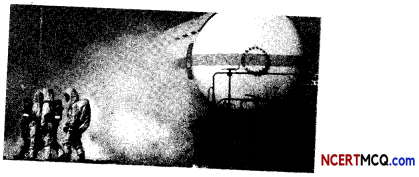What type of bond is present ammonia molecule? Wilt a solution of ammonia be a good conductor or poor conductor of electricity?
Ammonia molecule NH3 is formed by the sharing of electrons between nitrogen and hydrogen atoms as shown below:As electron pairs are shared between the three hydrogen atoms and the nitrogen atom, a solution of ammonia in water will be a poor conductor of electricity as there are no charged particles present in an ammonia molecule.Question 4.
The following table shows a part of the periodic table in which the elements are arranged according to their atomic numbers. (The letters given here are not the chemical symbols of the elements):(A) Which element has a bigger atom, a or f?
a (size decreases from left to right in a period).

(B) Which element has a higher valency, k or o?

(C) Which element is more metallic, i or k?
i (metallic character decreases from left to right in a period).

Explanation:it has to lose only one electron to acquire metallic character whereas k has to lose 3 electrons which requires a lot of energy as compared to element so element i is more metallic.

(D) Select a letter which represents a non-metal of valency 2.

Question 5.
When Sam was walking along the street, he saw several beautiful and colourful signages. In the signage industry, neon signs are electric signs lighted by long luminous gas-discharge tubes that contain rarefied neon or other gases. They are the most common use for neon lighting, which was first demonstrated in a modern form in December 1910 by Georges Claude at the Paris Motor Show.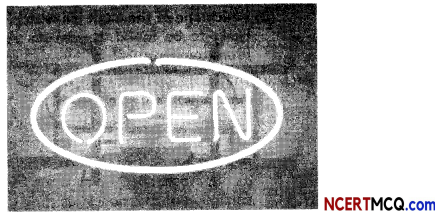Where would you locate the element with electronic configuration 2, 8 in the modern periodic table?
(a) Group 8
(b) Group 2
(c) Group 18
(d) Group 10
(c) Group 18
Explanation: The element with electronic configuration 2, 8 has an octet configuration, so it must be an inert gas, i.e., neon, which is placed in group 18.

Related Theory
Group 18 in the modern periodic table has noble gases (inert gases). Helium (He), neon (Ne), argon (Ar), krypton (Kr), xenon (Xe) and radon (Rn) are present in group 18. The members of the group have eight electrons in their outermost orbit (except helium which has two electrons). Thus, they have a stable electronic configuration. These gases are chemically un-reactive, i.e., they don’t react with other elements to form compounds.Question 6.
Atoms of eight elements A, B, C, D, E, F, G and H have the same number of electron shells but different number of electrons in their outermost shells. It was found that elements A and G combine to form an ionic compound. This ionic compound is added in a small amount to almost all vegetables and dishes during cooking. Oxides of elements A and B are basic in nature while those of elements E and F are acidic. The oxide of element D is, however, almost neutral.
Based on the above information, answer the following questions:
(A) To which group or period of the periodic table do these elements belong?

(B) What would be the nature of compound formed by a combination of elements B and F?
The compound between B and F will be ionic in nature.

(C) Which two of these elements could definitely be metals and which would be non metals?

(D) Which one of the eight elements is most likely to be found in gaseous state at room temperature?
Element H will be found in gaseous state at room temperature as it is the 8th element of the group so it would have 8 electrons in its outermost shell which is the electronic configuration of a nobel gas.

Related Theory:
Sodium chloride is used in almost all vegetables and dishes during cooking. It means A is sodium and G is chlorine.
Metals are one present on the left hand side of modern periodic table and non-metals on the right hand side. As we know oxides of metals are basic in nature and oxides of non-metals form acidic oxide. The elements given one.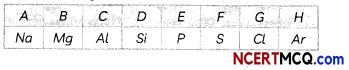Question 7.
Analyse the following table given below and answer the questions that follow:
Given alongside is a part of the periodic table. As we move vertically downward from Li to Fr:

 Li Be Na K Rb Cs Fr Ra

(A) Which among the following has larger size of atoms?
(a) Li
(b) Na
(c) Cs
(d) Fr
(d) Fr

Explanation: Fr has greater atomic size as the atomic size increases gradually from lithium to francium.

(B) Which one of the following does not increase while moving down in group of the periodic table?
(b) Metallic character
(c) Valence
(d) Number of shells in an element

(C) Name two properties of elements whose magnitude change while going from top to bottom in a group of the periodic table. In what manner do they change?

(D) Rewrite the following statement after correction, if necessary:
“Groups have elements with consecutive atomic numbers”
Periods have elements with consecutive atomic numbers.Question 8.
In addition to its applications in electronic devices, germanium is used as a component of alloys and in phosphors for fluorescent lamps. Because germanium is transparent to infrared radiation, it is employed in equipment used for detecting and measuring such radiation, such as windows and lenses. Silicon is used in building and construction, able to bond materials such as concrete, glass, granite, steel and plastics, enabling them to work better and last longer. Solar cells made out of silicon currently provide a combination of high effciency, low cost, and long lifetime.Three elements B, Si and Ge are:
(a) Metals
(b) Non-metals
(c) Metalloids
(d) Metal, non-metal and metalloid respectively
(c) Metalloids

Explanation: The elements boron (B), silicon (Si) and germanium (Ge) exhibit intermediate properties of metals and non-metals, therefore, they are called as metalloids. These elements are placed in between metals and non-metals diagonally as a zigzag line to separate them.

Related Theory
A few other elements are arsenic (As), antimony (Sb), tellurium (Te), polonium (Po) and astatine (At).

Question 9.
Analyse the following table given below and answer the questions that follow:
The atomic radii of the element of the second period are given below:(A) Arrange these elements in decreasing order of their atomic radii.
Elements in decreasing order of their atomic radii(B) Why does atomic radius decreases as we move from left to right in a period?

(C) Which is not true about the noble gases?
(a) They are non-metallic in nature
(b) They exist in atomic form
(c) They are radioactive in nature
(d) Xenon is the most reactive among these
(c) They are radioactive in nature.
Explanation: Noble gases are inert and are not radioactive in nature.

Related Theory
Noble gases cannot lose or gain or shore electrons as their outermost shell is complete.
He has 2 electrons in its K shell, which is completely filled as K shell can accommodate maximum of 2 electrons whereas Ne has 8 electrons in its L shell and its octet is complete so they exist in atomic form.

(D) Which of the following is the outermost shell for elements of period 2?
(a) K shell
(b) L shell
(c) M shell
(d) N shellQuestion 10.
A student tried to assign a correct position to hydrogen in Mendeleev’s Periodic Table by looking at its resemblance to alkali metals and the halogen family.
Another student wondered where she would place the isotopes of chlorine, Cl-35 and Cl-37 as they were atoms of the same element.

(A) No fixed position could be given to hydrogen in the Mendeleev’s Periodic Table as:
(I) Electronic configuration of hydrogen resembles that of alkali metals.
(II) Hydrogen combines with metals and non-metals to form ionic compounds.
(III) Hydrogen exists as diatomic molecules just like alkali metals.
(IV) Hydrogen combines with halogens, oxygen and sulphur to form compounds having similar formulae as alkali metals.
(a) Both (I) and (II)
(b) Both (II) and (III)
(c) Both (I) and (IV)
(d) Both (III) and (IV)
(c) Both (I) and (IV)

Explanation: As Mendeleev’s periodic table was based on atomic mass, no fixed position could be assigned to hydrogen. However, it has one valence electron just like alkali metals Li, Na, K etc. Moreover, hydrogen combines with haLogens, oxygen and sulphur just like alkali metals do. However, hydrogen combines with metals and non-metals to form covalent bonds by sharing of electrons and exists as diatomic molecules like halogens.

(B) The formula of hydrides of elements belonging to a Group of Mendeelev’s Periodic Table is RH2. The Group Number to which the element belongs is:
(a) Group IV
(b) Group V
(c) Group VI
(d) Group VII

(C) The formula of oxides and hydrides of elements belonging to different Groups of the Mendeleev’s Periodic Table is given below:The letter ‘R’ is used to represent any of the elements in the group.
Select the row containing incorrect information.
(a) Croup No.: 1, Formula of oxides; RO, Formula of Hydrides; RH Explanation: The formula of hydrides and oxides of elements belonging to group I of Mendeleev’s table is R2O and RH respectively. For example, formula of oxide and hydride of element Li will be Li2O and LiH respectively.

(D) Select the correct statement regarding position of isotopes in Mendeleev’s Periodic Table:
(a) Mendeleev left some gaps in his periodic table for the isotopes.
(b) Isotopes were discovered after Mendeleev had proposed his periodic classification of elements.
(c) Mendeleev placed both the isotopes of chlorine (Cl-35 and Cl-37) under the same slot as they had same chemical properties.
(d) Mendeleev placed both the isotopes of chlorine (Cl-35 and Cl-37) in different slots as they had different masses.

(E) Mendeleev left some gaps in his Periodic Table as he predicted the existence of some elements that had not been discovered at that time. Which of the following elements discovered later, have properties similar to Eka-boron?
(a) Gallium
(b) Germanium
(c) Caesium
(d) Scandium

Question 11.
We all have heard about the damage being done to the Taj Mahal and our environment due to high levels of oxides of certain elements in the atmosphere. Acid rain is caused by a chemical reaction that begins when these oxides are released into the air. These substances can rise very high into the atmosphere, where they mix and react with water, oxygen, and other chemicals to form more acidic pollutants, known as acid rain.Which of the following elements will form an acidic oxide?
(a) An element with atomic number 7
(b) An element with atomic number 3
(c) An element with atomic number 12
(d) An element with atomic number 19
(a) An element with atomic number 7

Explanation: Non-metals form acidic oxides. The element with atomic number 7 is a non-metal (nitrogen). Rest three elements with atomic numbers, 3 (Li), 12 (Mg) andl9 (K) are metals and hence, form basic oxides.Question 12.
Atomic radii of the elements of the second period are given below:
Period II elements: B Be O N Li C
Atomic radius (pm): 88 111 66 74 152 77
Atomic radii of first group elements are given below and arrange them in an increasing order.
Group 1 Elements 6: Na Li Rb Cs K
Atomic Radius (pm): 186 152 244 262 231
(A) Select the incorrect statements:
(I) The atomic radius decreases in moving from left to right along a period.
(II) The atomic radius increases in moving from left to right along a period
(III) As we move from left to right along a period, there is an increase in nuclear charge which tends to pull the electrons closer to the nucleus.
(IV) As we move from left to right along a period, there is a decrease in nuclear charge which tends to pull the electrons away from the nucleus.
(a) Both (I) and (III)
(b) Both (II) and (III)
(c) Both (I) and (IV)
(d) Both (II) and (IV)
(d) Both (II) and (IV)

Explanation: As we move from left to right along a period we see that the atomic radius decreases. This is due to an increase in nuclear charge which tends to pull the electrons closer to the nucleus and reduces the size of the atom.

(B) Two students recorded their observations on variation of atomic radii of first group elements as given below:

 First Student Second Student (a) Li has the smallest atomic radii Na has the smallest atomic radii (b) Atomic radius of Rb > Cs Atomic radius of Cs > Rb (c) Cs has the biggest atomic radius Li has the smallest atomic radius (d) Atomic radius of K > Na The atomic radius of Na > K

Select the row containing the correct observation by both students.
(c) First student: Cs has the biggest atomic radius, Second student: Li has the smallest atomic radius.

Explanation: The atomic radius and hence the atomic size increases as we move down a group. This is because a new shell is added as we move down the group. This increases the distance between the nucleus and the valence shell.

As Cs lies at the bottom of group 1, and Li lies at the top, Cs has the biggest atomic radius among Group 1 elements.

(C) The atomic size of Na is greater than Li because:
(a) Na has three shells, whereas Li has two shells in its atom
(b) The atomic number of Na is greater than Li
(c) As we go down a group, distance between outermost electrons and nucleus decreases.
(d) The mass of Na atom is greater than Li atom

(D) The graph of variation of atomic radius with atomic number for alkali metals and halogens is shown below: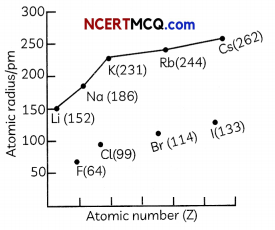Select the correct observation:
(a) Na has a bigger atomic radius than Li due to decrease in nuclear charge
(b) F has a smaller atomic radius than Li due to increase in nuclear charge
(c) Na and Cl belong to the third period of Modern Periodic Table and have identical electronic configuration.
(d) Elements of Group 17 have the biggest atomic size in their respective periods.
(b) F has a smaller atomic radius than Li due to increase in nuclear charge

Explanation: Both F and Li lie in the second period as they have two occupied shells. However, as the effective nuclear charge is more in the case of F as compared to Li, F has a smaller atomic radius than Li.

The atomic radius decreases in moving from left to right along a period and it increases in going from top to bottom along a group.

(E) The correct increasing order of the atomic radii of the elements Mg, Si and Cl is:
(a) Mg > Si > Cl
(b) Si < Cl < Mg
(c) Cl < Si < Mg
(d) Cl < Mg < SiQuestion 13.
Chemists have always looked for ways of arranging the elements to reflect the similarities between their properties. The earliest attempt to classify the elements was in 1789, when Antoine Lavoisier grouped the elements based on their properties into gases, non-metals, metals and earths. Several other attempts were made to group elements together over the coming decades. In 1829, Johann Dobereiner recognised triads of elements with chemically similar properties, such as lithium, sodium and potassium, and showed that the properties of the middle element could be predicted from the properties of the other two. It was not until a more accurate list of the atomic mass of the elements became available at a conference in Karlsruhe, Germany in 1860 that real progress was made towards the discovery of the modern periodic table.
(A) Which of the following elements form Dobereiner’s triads?
(a) Li, Na, K
(b) Ca, Sr, Ba
(c) F, Cl, Br
(d) Cl, Br, I

(B) Given below are four statements on Dobereiner’s triads:
(I) One triad identified by Dobereiner was (Ca, Na, Ba)
(III) The atomic mass of the middle element was roughly equal to the atomic masses of the other two elements.
(IV) Dobereiner could identify only three triads from the elements known at that time.
Identify the incorrect statements.
(a) Both (I) and (III)
(b) Both (II) and (III)
(c) Both (I) and (IV)
(d) Both (II) and (IV)
(a) Both (I) and (III)

Explanation: Dobereiner’s triods consisted of three elements having simiLar properties. He coutd identifij onLy three triads out of the elements discovered at that time. They were (Li, Na, K), (Ca. Sr. Ba) and (Cl, Br, I). The atomic mass of the middle element was roughly equal to the average of the atomic masses of the other two elements.

(C) A & B are two elements having similar properties which obey the law of octaves. How many elements are there in between A and B?
(a) 5
(b) 6
(c) 7
(d) 8

(D) Which of the following is not a limitation of Newland’s law of octaves:
(a) The elements fluorine, chlorine and bromine are placed in the same column (musical note).
(b) Newlands’ Law of Octaves worked well with lighter elements only.
(c) Newlands assumed that only 56 elements existed in nature and no more elements would be discovered in the future.
(d) In order to fit elements into his Table, Newlands adjusted cobalt and nickel in the same slot.
(a) The elements fluorine, chlorine and bromine are placed in the same column (musical note).

Explanation: The elements fluorine, chlorine and bromine are placed in the some column (musicol note) as they have similar properties and are known as halogens. Even in the modern periodic table, they are placed in the same group, group 17.

(E)Given below are few observations on properties of eLements.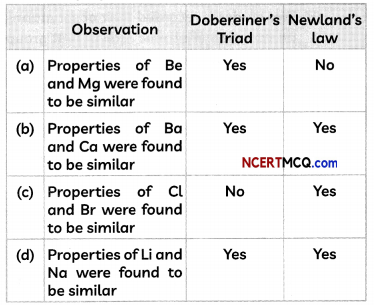Select the option containing the correct observation.
(d) Observation: Properties of Li and Na were found to be similar, Doberimer’s Triods: Yes, NewLands’ Law Yes.

Explanation: Both Be and Mg were placed under the same note in Newland’s law, but were not part of Döbereiner’s triads. Dobereiners TriadsQuestion 14.
Valency of an element is the combining capacity of an atom of the element. The volency of an element is related to how many electrons are in the outer shell. The chemical formula for a substance shows how many atoms of each element are present in a molecule, or the proportion of atoms of each element. The formula can be worked out using the volency.

The molecular formula of a compound can be predicted knowing the valency of the constituting atoms. Knowledge of valency is useful in calculating equivalent weight of elements, writing down chemical equations and in checking the structures of molecules. Some elements show variable valency and the valency determines the properties of elements. The table below gives atomic number of five elements written as P, Q, R, S and T, which are not their real chemical symbols.

 Element Atomic Number P 4 Q 9 R 14 S 18 T 20

(A) The valency and group number in Modern Periodic Table of four elements are given below:Select the option containing the correct valency and group number.

(B) The electronic configuration of the element T is:(C) The valency of elements:
(a) Decreases as we go down a group
(b) Remons same in a group
(c) Increases as we go down a group
(d) First increases and then decreases as we go down a group
(b) Remains same in a groupExplanation: The valency of elements is equal to the number of valence electrons in case of metals and (8 – valence electrons) for non-metals. Moreover, all elements belonging to the same group have same outer shell electronic configuration. Therefore, valency of elements remains same in a group.

(D) As per the table given above, the element(s) which wilL form only covaLent bonds ¡s:
(I) P
(II) R
(III) S
(IV) Q
(a) Only (I)
(b) Only (II)
(c) Both (I) and (IV)
(d) Both (II) and (III)

(E) Select the incorrect statement:
(a) Valency of elements of second period is 2.
(b) Valency of an element is determined by the number of valence electrons present in the outermost shell of its atom.
(c) Valency of metals is equal to the number of valence electrons.
(d) Valency of elements of Group 18 of Modern Periodic Table is 0.Question 15.
The elements can be classified as metals, nonmetals, or metalloids. In the Modern Periodic Table, a zig-zag line separates metals from non-metals.The metals are to the Left of the line (except for hydrogen, which is a nonmetaL), the nonmetals are to the right of the Line, and the elements immediately adjacent to the line are the metalioids.
Ionization energy is the minimum amount of energy required to remove the most loosely bound electron of an isolated neutral gaseous atom or molecule. It is expressed as
X(g) + energy → X+(g) + e

where X is any atom or molecule, X+ is the ion with one electron removed, and e- is the removed electron.
Since the metals are further to the left on the periodic table, they have low ionization energies.
(A) Which of the following elements is not a metalloid?
(a) Si
(b) Ge
(c) As
(d) Ar
(d)Ar

Explanation: The metalloids are B, Si. Ge, As, Te and Po. Ar (Argon) is a noble gas having atomic number 18.

(B) An element A (atomic number 17) reacts with an element B (atomic number 14) to form a tetravalent halide.
Select the option which gives the correct nature of elements A and B:

 Element A Element B (a) Metal Non-metal (b) Non-metal Non-metal (c) Non-metal Metalloid (d) Metalloid Metalloid

(C) Four statements are given below.
(I) The metalloids are placed in Groups 13 – 16 in the Modern Periodic Table
(II) Boron is the only metalloid in group 13 of the Modern Periodic Table.
(III) Group 14 of the Modern Periodic Table contains only metalloids.
(IV) Group 17 of the Modern Periodic Table contains both metalloids and non-metals
Select the correct statement(s):
(a) Both (I) and (II)
(b) Both (I) and (III)
(c) Both (II) and (IV)
(d) (I), (II) and (IV)

(D) Variation of the ionization energy of elements with atomic number upto atomic number 20 is given below:
From the graph we can conclude that the tendency to lose electrons:
(a) Increases as we go down the group
(b) Decreases as we go down the group
(c) Remains constant in a group
(d) No definite conclusion can be made

(E) The metalloid which is the second most abundant element on earth’s crust after oxygen and is widely used in electronics is:
(a) B (b) Ge
(c) Si (d) As

Question 1.
State the common characteristic of the following elements: Boron, Silicon, Germanium and ArsenicQuestion 2.
State the Periodic Law on which the Modern Periodic Table is based.
Periodic Law on which the Modern Periodic Table is based is termed as Modern Periodic Law. According to Modern Periodic Law – The properties of elements are a periodic function of their atomic numbers.

Related Theory
Modern Periodic Table or Long form of Periodic table was prepared by Henry Moseley and is based on electronic configuration of elements. The elements are arranged in increasing order of their atomic numbers. Modern Periodic Table consists of 18 vertical columns called 7 horizontal rows called periods.

Question 3.
How many metals are present in second period of periodic table?

Question 4.
The atomic radii of three elements A, B and C of a periodic table are 186 pm, 104 pm and 143 pm respectively. Giving a reason, arrange these elements in the increasing order of atomic numbers in the period.
Since atomic size decreases along a period and the atomic number increases. So, the element with smaller radii, has the highest atomic number.

Hence, B has the highest atomic number followed by C and A i.e. A < C < B

Explanation: The atomic size is the distance between the centre of the nucleus and the outermost shell of an isolated atom. The atomic radius is measured in picometre, (1 pm = 10-12 m).

The atomic radius decreases in moving from left to right along a period. This is due to:
As the atomic number increases, the nuclear charge increases. Increase in nuclear charge tends to pull the electrons closer to the nucleur and reduce the size of the atom.Question 5.
Consider the following :
20Ca, 8O,16S, 4Be
Which of the above elements would you expect to. be in group 2 of the Modern Periodic table?
Consider the electronic configuration of elements:
Ca: 2, 8, 8, 2 O: 2, 6 S: 2, 8, 6 Be: 2, 2
As Ca and Be all have the same number of valence electrons (2), they belong to group 2 of the Modern Periodic Table.

Question 6.
Define electropositivity.
Electropositivity is the ability metals to lose electron to form positive ions.
Explanation: Metals have 1, 2, 3 valence electrons and have a strong tendency to lose these to form positive ions, so metal are called electropositive elements.
For example: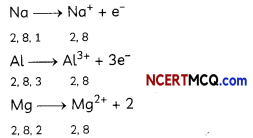as we move down in a group in the periodic table, atomic size increases. As a result, force of attraction between the nucleus and valence electrons decreases.

Question 7.
Write two reasons responsible for the late discovery of noble gases